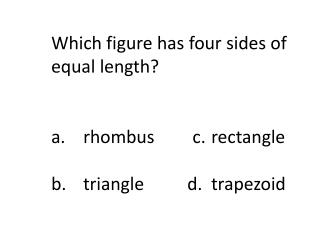# Which figure has four sides of equal length? a. rhombus c. rectangle - PowerPoint PPT PresentationDownload PresentationWhich figure has four sides of equal length? a. rhombus c. rectangle

Which figure has four sides of equal length? a. rhombus c. rectangleDownload Presentation## Which figure has four sides of equal length? a. rhombus c. rectangle

- - - - - - - - - - - - - - - - - - - - - - - - - - - E N D - - - - - - - - - - - - - - - - - - - - - - - - - - -
##### Presentation Transcript

1. Which figure has four sides of equal length? a. rhombus c. rectangle b. triangle d. trapezoid

2. What kind of angle is angle M?

3. This shows a line plot of Mrs. Smith’s class. It shows that 18 Students got a C or higher.

4. Angles On One Side of A Straight Line Angles on one side of a staight line will always add to 180 degrees. If a line is split into 2 and you know one angle you can always find the other one. 30° + 150° = 180° Angles On One Side of A Straight Line Angles on one side of a staight line will always add to 180 degrees.

5. AREA is The size of a surface.The amount of space inside the boundary of a flat (2-dimensional) object such as a triangle or circle.These areas are all the same (9).